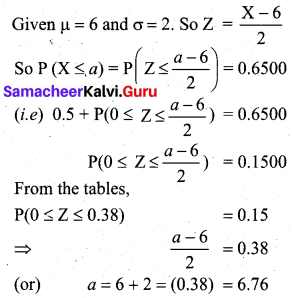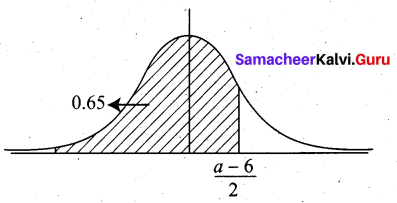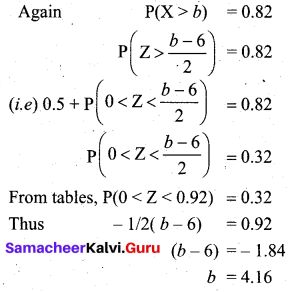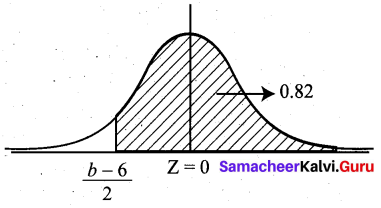# Samacheer Kalvi 12th Business Maths Solutions Chapter 7 Probability Distributions Additional Problems

Question 1.
If X is a poisson variate with P (X = 1) = P (X = 2), the mean of the poisson variate is equal to _____
(a) 1
(b) 2
(c) -2
(d) 3
(b) 2
Hint:
P(X = 1) = P(X = 2)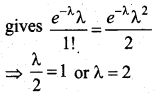Question 2.
If ‘λ’ is the mean of the Poisson distributions, then P (X = 0) is given by ________
(a) $$e^{-\lambda}$$
(b) $$e^{\lambda}$$
(c) e
(d) $$\lambda^{-e}$$
(a) $$e^{-\lambda}$$
Hint: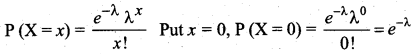Question 3.
A larger standard deviation for a normal distribution with an unchanged mean indicates that the curve becomes, ____
(a) narrower and more peaked
(b) flatter and wider
(c) more skewed to the right
(d) more skewed to the left
(b) flatter and wider

Question 4.
If X ~ N (0, 4), the value of P(|X| ≥ 2.2) is ______
(a) 0.2321
(b) 0.8438
(c) 0.2527
(d) 0.2714
(d) 0.2714
Hint:
P (|X| ≥ 2.2)
= P (X > 2.2) + P (X < -2.2)
= P(Z > $$\frac{2.2-0}{2}$$) + P(Z < $$\frac{-2.2-0}{2}$$)
= P(Z > 1.1) + P(Z < -1.1)
= 2 P(Z > 1.1) (by symmetry)
= 2[0.5 – P(0 < Z < 1.1)]
= 2 [0.5 – 0.3643]
= 0.2714Question 5.
For a binomial distribution.

1. If n = 1, then E(X) is ________
2. The variance is always ________
3. Successive trials are ______
4. Negatively skewed when _______
5. Number of parameters is _______
6. n = 10, p = 0.3, variance is _______
7. Is symmetrical when ______
8. n = 6, p = 0.9, P (X = 7) is _______
9. Mean, median and mode will be equal when ______

1. p
2. less than mean
3. independent
4. p > $$\frac {1}{2}$$
5. two
6. 2.1
7. p = q
8. zero
9. p = 0.5

Question 6.
For a Normal distribution

1. The parameters which controls the flatness of the curve is _____ & ______
2. If Y = 5X + 10 and X ~ N (10, 25), then mean of Y is _______
3. Normal curve is asymptotic to the ________
4. The median corresponds to the value of Z = _______
5. The area under the normal curve on either side of mean is _______

1. µ, σ
2. 60
3. X-axis
4. µ
5. 0.5Question 7.
Heights of college girls follows a normal distribution with mean 65 inches and a standard deviation of 3 inches. About what proportion of girls are between 65 and 67 inches tall?
(a) 0.75
(b) 0.5
(c) 0.25
(d) 0.17
(c) 0.25
Hint:
P (65 < X < 67)
= P($$\frac{65-65}{3}$$ < Z < $$\frac{67-65}{3}$$)
= P(0 < Z < 67)
= 0.2486 ~ 0.25

Question 8.
Which one of these is a binomial random variable?
(a) time is taken by a randomly chosen student to complete an exam
(b) number of books bought by a randomly chosen student
(c) number of women taller than 150 cm in a random sample of 10 women
(d) number of CD’s a randomly selected person owns
(c) number of women taller than 150 cm in a random sample of 10 womenQuestion 9.
Pulse rates of adult men follows a normal distribution with a mean of 70 and a standard deviation of 8. Which choice tells how to find the proportion of men that have a pulse rate greater than 78?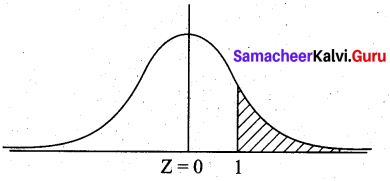(a) find the area to the left of Z = 1 under the normal curve
(b) find the area between Z = -1 and Z = 1 under the standard normal curve
(c) find the area to the right of Z = 1 under the standard normal curve
(d) find the area to the right of Z = -1 under the standard normal curve
(c) find the area to the right of Z = 1 under the standard normal curve
Hint:
P(X > 78) = P(Z > $$\frac{78-70}{8}$$) = P(Z > 1)

2 Mark Questions

Question 1.
The probability that a radio manufactured by a company will be defective is $$\frac{1}{10}$$. If 15 such radios are inspected, find the probability that exactly 3 will be defective.
Solution:
Given n = 15, p = $$\frac{1}{10}$$ = 0.1, q = 0.9 10
Let X be the binomial variable, denoting the number of radios.
We want probability of exactly 3 defectives, (i.e) P (X = 3)
Now P (X = 3) = $$^{15} \mathrm{C}_{3}(0.1)^{3}(0.9)^{12}$$
= 455 (0.001) [(0.9)4]3
= (455) (0.001) (0.6561)3
= 0.1285

Question 2.
The probability that a bulb produced in a factory will fuse after 10 days is 0.05. Find the probability that out of 5 such bulbs, not more than 1 will fuse after 400 days of use.
Solution:
Given that X ~ B (5, 0.05)
(i.e.) n = 5, p = 0.05, q = 0.95
P(X ≤ 1) = P(X = 0) + P(X = 1)
= $$^{5} \mathrm{C}_{0}(0.05)^{0}(0.95)^{5}+^{5} \mathrm{C}_{1}(0.05)^{1}(0.95)^{4}$$
= (0.95)5 + 5 (0.05) (0.95)4
= (0.95)4 + [0.95 + 0.25]
= 0.9774Question 3.
The number of traffic accidents that occur in a particular road follows a Poisson distribution with a mean of 9.4. Find the probability that less than two accidents will occur on this road during a randomly selected month.
Solution:
Let X be the Poisson variate with mean λ = 9.4.
Now P(X < 2) = P (X = 0) + P (X = 1)
= $$e^{-9.4}$$ [1 + 9.4]
= $$e^{-9.4}$$ (10.4)
= 0.00086

Question 4.
What is the probability of getting 2 Sundays out of 15 days selected at random?
Solution: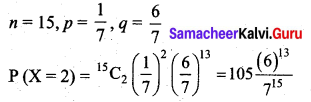Question 5.
Find the mean and standard deviation of a Poisson variate X which satisfies the condition P(X = 2 ) = P(X = 3)
Solution:
P(X = 2 ) = P(X = 3)
$$\frac{e^{-\lambda} \lambda^{2}}{2 !}=\frac{e^{-\lambda} \lambda^{3}}{3 !}$$
1 = $$\frac{\lambda}{3}$$ (or) λ = 3
Variance for Poisson distribution is λ = 3. Hence s.d = √3

Question 6.
Between 5 to 6 p.m., the average number of phone calls per minute is 4. What is the probability that there is no phone call during a minute?
Solution:
Let X be the Poisson variate denoting the number of phone calls per minute.
Given that mean λ = 4. We want P(X = 0)
Now P(X = 0) = $$\frac{e^{-4} \lambda^{0}}{0 !}$$ = e-4 = 0.0183Question 7.
2% cars are defective. What is the probability that one of 150 cars, there is exactly one defective car?
Solution:
Let X be the Poisson variate denoting the number of defective cars. The mean λ is given by
λ = $$\frac {2}{100}$$ × 150 = 3
So P(X = 1) = $$\frac{e^{-3}(3)^{1}}{1 !}$$ = 3e-3 = 0.1494

Question 8.
What is the standard deviation of the number of recoveries among 48 patients, the probability of recovering is 0.75.
Solution:
Given n = 48, p = 0.75, q = 0.25
This is a binomial distribution.
The variance is given by Var (X) = npq
= (48) (0.75) (0.25)
= 9 .
So the standard deviation is √9 = 3

3 and 5 Mark Questions

Question 1.
What is the probability of success of the binomial distribution satisfying the following condition: 4P(X = 4) = P(X = 2) and having other parameter as 6?
Solution:
Given n = 6, 4P(X = 4) = P(X = 2).
We have to find p.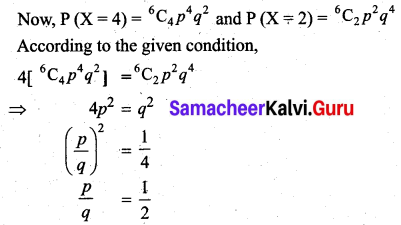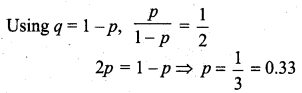Question 2.
Wool fibre breaking strengths are normally distributed with mean µ = 23.56 and S.D σ = 4.55. What proportion of fibres would have a breaking strength of 14.45 or less?
Solution: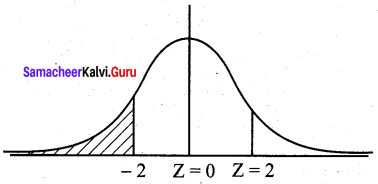Let X be the normal variable denoting the breaking strengths.
We want to find
P(X ≤ 14.45) = P(Z ≤ $$\frac{14.45-23.56}{4.55}$$) = P(Z ≤ -2.00)
By Symmetry,
P(Z ≤ -2) = P(Z ≥ 2)
= 0.5 – P(0 ≤ Z ≤ 2)
= 0.5 – 0.4772
= 0.0228
Hence the proportion of fibres with a breaking strength of 14.45 or less is 2.28%Question 3.
The finish times for marathon runners during a race are normally distributed with a mean of 195 minutes and a standard deviation of 25 minutes.
(a) What is the probability that a runner will complete the marathon within 3 hours?
(b) Calculate to the nearest minute, the time by which the first 8% runners have completed the marathon?
(c) What proportion of the runners will complete the marathon between 3 hours and 4 hours?
Solution:
Let X be the normal variate denoting the finish time of the marathon runners.
Given the mean µ = 195 and s.d σ = 25
(a) P(X ≤ 3 hours)
= P(X ≤ 180 minutes)
= P(Z ≤ $$\frac{180-195}{25}$$)
= P(Z ≤ -0.6)
By symmetry
P(Z ≤ -0.6)
= P(Z ≤ 0.6)
= 0.5 – P(0 ≤ Z ≤ 0.6)
= 0.5 – 0.2258
= 0.2742
(i.e) Probability of a runner taking less than 3 hours is 0.2742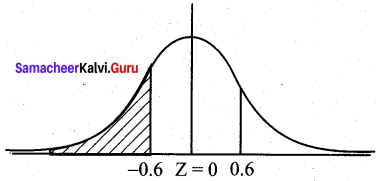(b) Given the probability is 8% = 0.08
P (Z ≥ z) = 0.08
0.5 – P(0 ≤ Z ≤ z) = 0.08
P(0 ≤ Z ≤ z) = 0.42
z = 1.41 (from normal tables)
Hence $$\frac{X-195}{25}$$ = -1.41
X = 25 (-1.41) + 195 = 159.75 = 160 minutes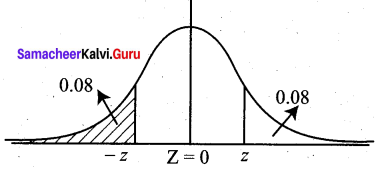(c) P(3 hours < X < 4 hours)
= P (180 min < X < 240 min)
= P($$\frac{180-195}{25}$$ < Z < $$\frac{240-195}{25}$$)
= P(-0.6 < Z < 1.8)
= P (-0.6 < Z < 0) + P (0 < Z < 1.8)
= P(0 < Z < 0.6) + P(0 < Z < 1.8)
= 0.2258 + 0.4641
= 0.6899
Hence the proportion of runners taking between 3 hours and 4 hours is 68.99%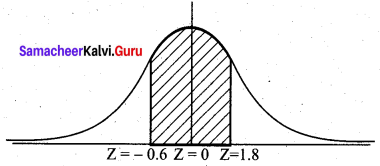Question 4.
The probability that a driver must stop at any one traffic light is 0.2. There are 15 sets of traffic lights on the journey.
(а) What is the probability that a student must stop at exactly 2 of the 15 sets of traffic lights?
(b) What is the probability that a student will be stopped at 1 or more of the 15 sets of traffic lights?
Solution:
Let X be the binomial random variable denoting the number of traffic lights.
Given n = 15, p =0.2, q = 0.8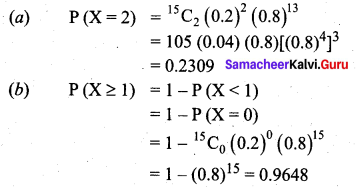Question 5.
A radioactive source emits 4 particles on average during a five-second period.
(а) Calculate the probability that it emits 3 particles during a five-second period.
(b) Find the probability that it emits at least one particle during a 5 second period.
(c) During a 10 second period, what is the probability that 6 particles are emitted?
Solution:
Let X be the Poisson variable denoting the number of particles emitted.
Given the mean λ = 4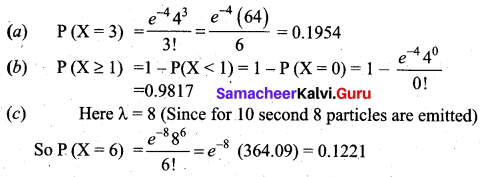Question 6.
Given that X ~ B (n, p) and E(X) = 24, Var(X) = 8, find the values of n and p.
Solution:
We know E(X) = np = 24 and Var(X) = npq = 8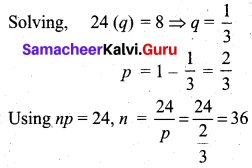Question 7.
Given that X ~ N (6, 4), find the values of ‘a’ and ‘b’ such that P (X ≤ a) = 0.6500 and P(X ≤ b) = 0.8200
Solution: